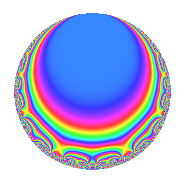Properties

 Label 37.2.eLevel 37 Weight 2 Character orbit e Rep. character $$\chi_{37}(11,\cdot)$$ Character field $$\Q(\zeta_{6})$$ Dimension 4 Newforms 1 Sturm bound 6 Trace bound 0

Related objects

Defining parameters

 Level: $$N$$ = $$37$$ Weight: $$k$$ = $$2$$ Character orbit: $$[\chi]$$ = 37.e (of order $$6$$ and degree $$2$$) Character conductor: $$\operatorname{cond}(\chi)$$ = $$37$$ Character field: $$\Q(\zeta_{6})$$ Newforms: $$1$$ Sturm bound: $$6$$ Trace bound: $$0$$

Dimensions

The following table gives the dimensions of various subspaces of $$M_{2}(37, [\chi])$$.

Total New Old
Modular forms 8 8 0
Cusp forms 4 4 0
Eisenstein series 4 4 0

Trace form

 $$4q$$ $$\mathstrut +\mathstrut 2q^{3}$$ $$\mathstrut -\mathstrut 2q^{4}$$ $$\mathstrut -\mathstrut 6q^{5}$$ $$\mathstrut -\mathstrut 2q^{9}$$ $$\mathstrut +\mathstrut O(q^{10})$$ $$4q$$ $$\mathstrut +\mathstrut 2q^{3}$$ $$\mathstrut -\mathstrut 2q^{4}$$ $$\mathstrut -\mathstrut 6q^{5}$$ $$\mathstrut -\mathstrut 2q^{9}$$ $$\mathstrut -\mathstrut 8q^{10}$$ $$\mathstrut -\mathstrut 12q^{11}$$ $$\mathstrut +\mathstrut 2q^{12}$$ $$\mathstrut +\mathstrut 12q^{13}$$ $$\mathstrut +\mathstrut 6q^{15}$$ $$\mathstrut +\mathstrut 2q^{16}$$ $$\mathstrut +\mathstrut 6q^{17}$$ $$\mathstrut +\mathstrut 12q^{18}$$ $$\mathstrut -\mathstrut 6q^{19}$$ $$\mathstrut +\mathstrut 6q^{20}$$ $$\mathstrut +\mathstrut 12q^{21}$$ $$\mathstrut +\mathstrut 6q^{22}$$ $$\mathstrut -\mathstrut 18q^{24}$$ $$\mathstrut +\mathstrut 4q^{25}$$ $$\mathstrut -\mathstrut 16q^{27}$$ $$\mathstrut +\mathstrut 2q^{30}$$ $$\mathstrut -\mathstrut 12q^{33}$$ $$\mathstrut -\mathstrut 4q^{34}$$ $$\mathstrut -\mathstrut 24q^{35}$$ $$\mathstrut +\mathstrut 4q^{36}$$ $$\mathstrut +\mathstrut 2q^{37}$$ $$\mathstrut -\mathstrut 12q^{38}$$ $$\mathstrut +\mathstrut 12q^{39}$$ $$\mathstrut +\mathstrut 12q^{40}$$ $$\mathstrut -\mathstrut 6q^{41}$$ $$\mathstrut -\mathstrut 12q^{42}$$ $$\mathstrut +\mathstrut 6q^{44}$$ $$\mathstrut -\mathstrut 2q^{46}$$ $$\mathstrut +\mathstrut 12q^{47}$$ $$\mathstrut +\mathstrut 4q^{48}$$ $$\mathstrut -\mathstrut 10q^{49}$$ $$\mathstrut +\mathstrut 24q^{50}$$ $$\mathstrut -\mathstrut 12q^{52}$$ $$\mathstrut +\mathstrut 12q^{53}$$ $$\mathstrut +\mathstrut 6q^{55}$$ $$\mathstrut +\mathstrut 36q^{56}$$ $$\mathstrut +\mathstrut 12q^{57}$$ $$\mathstrut -\mathstrut 4q^{58}$$ $$\mathstrut -\mathstrut 12q^{59}$$ $$\mathstrut -\mathstrut 6q^{61}$$ $$\mathstrut +\mathstrut 6q^{62}$$ $$\mathstrut +\mathstrut 48q^{63}$$ $$\mathstrut -\mathstrut 28q^{64}$$ $$\mathstrut -\mathstrut 12q^{65}$$ $$\mathstrut +\mathstrut 18q^{67}$$ $$\mathstrut -\mathstrut 12q^{70}$$ $$\mathstrut -\mathstrut 12q^{71}$$ $$\mathstrut -\mathstrut 36q^{72}$$ $$\mathstrut -\mathstrut 40q^{75}$$ $$\mathstrut +\mathstrut 6q^{76}$$ $$\mathstrut +\mathstrut 12q^{77}$$ $$\mathstrut -\mathstrut 12q^{78}$$ $$\mathstrut -\mathstrut 18q^{79}$$ $$\mathstrut -\mathstrut 2q^{81}$$ $$\mathstrut +\mathstrut 6q^{83}$$ $$\mathstrut -\mathstrut 24q^{84}$$ $$\mathstrut +\mathstrut 4q^{85}$$ $$\mathstrut +\mathstrut 6q^{87}$$ $$\mathstrut +\mathstrut 18q^{89}$$ $$\mathstrut -\mathstrut 8q^{90}$$ $$\mathstrut +\mathstrut 6q^{92}$$ $$\mathstrut -\mathstrut 36q^{93}$$ $$\mathstrut +\mathstrut 18q^{94}$$ $$\mathstrut +\mathstrut 18q^{95}$$ $$\mathstrut +\mathstrut 30q^{96}$$ $$\mathstrut +\mathstrut 18q^{99}$$ $$\mathstrut +\mathstrut O(q^{100})$$

Decomposition of $$S_{2}^{\mathrm{new}}(37, [\chi])$$ into irreducible Hecke orbits

Label Dim. $$A$$ Field CM Traces $q$-expansion
$$a_2$$ $$a_3$$ $$a_5$$ $$a_7$$
37.2.e.a $$4$$ $$0.295$$ $$\Q(\zeta_{12})$$ None $$0$$ $$2$$ $$-6$$ $$0$$ $$q+\zeta_{12}q^{2}+(-\zeta_{12}+\zeta_{12}^{2}-\zeta_{12}^{3})q^{3}+\cdots$$